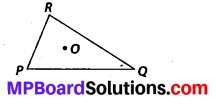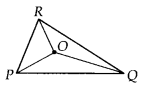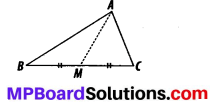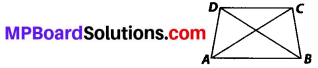# MP Board Class 7th Maths Solutions Chapter 6 The Triangles and Its Properties Ex 6.4

## MP Board Class 7th Maths Solutions Chapter 6 The Triangles and Its Properties Ex 6.4

Question 1.
Is it possible to have a triangle with the following sides?
(i) 2 cm, 3 cm, 5 cm
(ii) 3 cm, 6 cm, 7 cm
(iii) 6 cm, 3 cm, 2 cm
Solution:
In a triangle, the sum of the lengths of either two sides is always greater than the third side.
(i) Given that, the sides of the triangle are 2 cm, 3 cm, 5 cm.
It can be observed that,
(2 + 3) cm = 5 cm
However, 5 cm = 5 cm
Hence, this triangle is not possible.

(ii) Given that, the sides of the triangle are
3 cm, 6 cm, 7 cm.
Here, (3 + 6) cm = 9 cm > 7 cm
(6 + 7) cm = 13 cm > 3 cm
(3 + 7) cm = 10 cm > 6 cm
Hence, this triangle is possible.

(iii) Given that, the sides of the triangle are
6 cm, 3 cm, 2 cm.
Here, (6 + 3) cm = 9 cm > 2 cm
However, (3 + 2) cm = 5 cm < 6 cm Hence, this triangle is not possible.

Question 2.
Take any point 0 in the interior of a triangle PQR. Is(i) OP + OQ > PQ?
(ii) OQ + OR > QR?
(iii) OR + OP > RP?
Solution:
If O is a point in the interior of a given triangle, then three triangles ∆OPQ, ∆OQR and ∆ORP can be constructed. In a triangle, the sum of the lengths of either two sides is always greater than the third side.
(i) Yes, as OQR is a triangle with sides OR, OQ and QR.∴ OQ + OR> QR

(ii) Yes, as OQR is a triangle sides OR, OQ and QR.
∴ OQ + OR > QR

(iii) Yes, as A ORP is a triangle with sides OR, OP and PR.
∴ OR + OP > PR

Question 3.
AM is a median of a triangle ABC.
Is AB + BC + CA > 2AM?
(Consider the sides of ∆ABM and ∆AMC.)Solution:
In a triangle, the sum of the lengths of either two sides is always greater than the third side.
In ∆ABM,
AB + BM > AM …(i)
Similarly, in ∆ACM,
AC + CM > AM …(ii)
Adding (i) and (ii), we obtain
AB + BM + MC +AC > AM + AM
AB + BC + AC > 2AM
Yes, the given expression is true.

Question 4.Is AB + BC + CD + DA > AC + BD ?
Solution:
In a triangle, the sum of the lengths of either two sides is always greater than the third side.
In ∆ABC; AB + BC > CA …(i)
In ∆BCD; BC + CD > DB …(ii)
In ∆CDA; CD + DA > AC …(iii)
In ∆DAB; DA + AB > DB …(iv)
Adding (i), (ii), (iii) and (iv), we obtain
AB + BC + BC + CD + CD + DA + DA+ AB > AC + BD + AC + BD
⇒ 2AB + 2BC + 2CD + 2DA > 2AC + 2BD ⇒ 2{AB + BC + CD + DA) > 2(AC+BD)
⇒ (AB + BC + CD + DA) > (AC + BD)
Yes, the given expression is true.

Question 5.Is AB + BC + CD + DA < 2(AC + BD)?
Solution:
In a triangle, the sum of the lengths of either two sides is always greater than the third side.
Let diagonals AC and BD of the quadrilateral ABCD intersects at O.In ∆OAB, OA + OB > AB … (i)
In ∆OBC, OB + OC > BC … (ii)
In ∆OCD, OC + CD > CD … (iii)
In ∆ODA, OD + OA > DA … (iv)
OA + OB + OB + OC + OC + OD + OD + OA > AB + BC + CD + DA
⇒ 20A + 20B + 20C + 20D > AB + BC + CD + DA
⇒ 2(OA + OC) + 2(OB + OD) > AB + BC + CD + DA
⇒ 2(AC) + 2(BD) > AB + BC + CD + DA
⇒ 2(AC + BD) > AB + BC + CD + DA
Yes, the given expression is true.

Question 6.
The lengths of two sides of a triangle are 12 cm and 15 cm. Between what two measures should the length of the third side fall?
Solution:
In a triangle, the Sum of the lengths of either two side is always greater than the third side.
Lengths of two sides of a triangle are 12 cm and 15 cm.
Let the third side be x cm.
∴ x + 12 > 15 ⇒ x > 3
x + 15 > 12 ⇒ x > – 3 but side length never be negative.
and 12 + 15 > x ⇒ 27 > x
Hence, third side can measure between 3 and 27.